Reach Us+44-1522-440391
On Nonparametric Hazard Estimation | OMICS International
Journal of Biometrics & Biostatistics

Like us on:

All submissions of the EM system will be redirected to Online Manuscript Submission System. Authors are requested to submit articles directly to Online Manuscript Submission System of respective journal.

# On Nonparametric Hazard Estimation

Hobbs BP*

Department of Biostatistics, University of Texas M.D. Anderson Cancer Center, Houston, TX, USA

*Corresponding Author:
Hobbs BP
Department of Biostatistics, University of Texas M.D. Anderson Cancer Center
Houston, TX, USA
Tel: 713-794-4633
E-mail: [email protected]

Received date: May 29, 2015; Accepted date: June 10, 2015; Published date: June 18, 2015

Citation: Hobbs BP (2015) On Nonparametric Hazard Estimation. J Biomet Biostat 6:232. doi: 10.4172/2155-6180.1000232

Copyright: © 2015 Hobbs BP. This is an open-access article distributed under the terms of the Creative Commons Attribution License, which permits unrestricted use, distribution, and reproduction in any medium, provided the original author and source are are credited.

Visit for more related articles at Journal of Biometrics & Biostatistics

#### Abstract

The Nelson-Aalen estimator provides the basis for the ubiquitous Kaplan-Meier estimator, and therefore is an essential tool for nonparametric survival analysis. This article reviews martingale theory and its role in demonstrating that the Nelson-Aalen estimator is uniformly consistent for estimating the cumulative hazard function for right-censored continuous time-to-failure data.

#### Keywords

Asymptotic theory; Counting process; Hazard estimation; Martingale theory; Nelson-Aalen estimator; Time-to-failure analysis

#### Introduction

The Nelson-Aalen estimator Nelson [1,2] provides the foundation for the ubiquitous Kaplan-Meier survival estimator which consists of its product-integral. This article reviews martingale theory and its role in demonstrating that the Nelson-Aalen estimator is asymptotically uniformly consistent for the cumulative hazard function for rightcensored continuous time-to-failure data and demonstrates its application using simulation.

#### Fundamental Principles

Nonparametric statistical models for censored data were developed using counting processes which decompose into a martingales and integrated intensity processes. Martingale methods facilitate direct evaluation of small and large sample properties of hazard estimators for right censored failure time data. This section provides formal definition of a martingale process as well as reviews several intrinsic properties that are used to study small and large sample properties of hazard estimators for right censored failure time data.

Counting processes

A family of sub-σ-algebras {Ft: t ≥ 0} of a σ-algebra F is called increasing if s ≤ t implies Fs⊂ Ft. An increasing family of sub-σ-algebras is called a filtration. When {Ft: t ≥ 0} is a filtration, the σ-algebra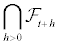is usually denoted by Ft+. The corresponding limit from the left, Ft-, is the smallest σ-algebra containing all the sets in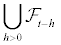and is written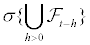. A filtration {Ft: t ≥ 0} is right-continuous if, for any t, Ft+=Ft. A stochastic basis is a probability space (Ω, F, P) with a rightcontinuous filtration {Ft: t ≥ 0}, and is denoted by (Ω, F,{Ft: t ≥ 0}, P). A stochastic process {X(t): t ≥ 0} is adapted to a filtration if, for every t ≥ 0, X (t) is Ft-measurable. A counting process is a stochastic process {N (t): t ≥ 0} adapted to a filtration {Ft: t ≥ 0} with N (0)=0 and N (t)<∞ almost surely (a.s.), and whose paths are with probability one rightcontinuous, piecewise constant, and have only jump discontinuities with jumps of size +1. If N is a counting process, f is some function of time, and 0 ≤ s<t ≤ ∞, then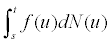f u dN u , is the Stieltjes integral representation of the sum of the values of f at the jump times of N n the interval (s, t].

Martingales

Let X={X(t): t ≥ 0} denote a right-continuous stochastic process with left-hand limits and {Ft: t ≥ 0} a filtration, defined on a common probability space. X is called a martingale with respect to {Ft: t ≥ 0} if, X is adapted to {Ft: t ≥ 0}, E|X (t)|<∞ for all t<∞, and E[X (t+s)|Ft]=X (t) a.s. for all s ≥ 0, t ≥ 0. Thus, a martingale is essentially a process that has no drift and whose increments are uncorrelated. If E[X (t+s)|Ft] ≥ X (t) a.s. X is a submartingale. Two fundamental properties of martingales are, for any h>0,

E[X (t)|Ft-h]=X (t-h), and

E[X (t)-X (t-h)|Ft-h]=X (t-h)-X (t-h)=0.

Predictable processes

The stochastic process X is said to be predictable with respect to filtration Ft if for each t, the value of X(t) is specified by Ft- and therefore is Ft--measurable (Kalbfleisch and Prentice, 2002). Theorem 7.1 in Appendix A is the version of the Doob-Meyer Decomposition Theorem provided by (Fleming and Harrington, 1991), which states that for any right-continuous nonnegative submartingale X there is a unique increasing right-continuous predictable process A such that A(0)=0 and X-A is a martingale. Also, there is a unique process A so that for any counting process N with finite expectation, N-A is a martingale. This is shown in the Corollary 7.2 . The process A in Corollary 7.2 of Appendix A is referred to as the compensator for the submartingale X.

Square integrable martingales

A martingale, X(t) is called square integrable if E[X2(t)]=var[X(t)]<∞ for all t ≤ τ, or equivalently, if E[X2(τ )]<∞ . The variance of a squareintegrable martingale X(t) is estimated using the predictable variation process,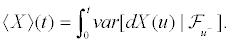For martingales Xi, Xj,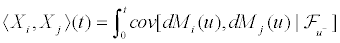is the predictable covariation process of Xi and Xj. If (Xi, Xj)(t)=0 for all t, then Xi and Xj are orthogonal martingales (Fleming and Harrington, 1991). Suppose Xi, Xj are orthogonal martingales, for all i ≠ j . Then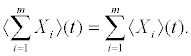Localization

A nonnegative random variable τ is a stopping time with respect to filtration {Ft} if {τ ≤ t} ∈ Ft for all t ≥ 0. An increasing sequence of random times τm, m=1, 2, ..., is a localizing sequence with respect to a filtration if each τm is a stopping time and limm→∞ τm=∞ (Fleming and Harrington, 1991). A stochastic process X={X(t): t ≥ 0} is a local martingale (submartingale) with respect to a filtration {Ft: t ≥ 0} if there exists a localizing sequence {τn} such that, for each n, Xn={X (t ∧ τn): 0 ≤ t<∞} is an Ft-martingale (submartingale). If Xn is a martingale and a square integrable process, Xn is a square integrable martingale and X is called a local square integrable martingale. It can be shown that any martingale is a local martingale by simply taking τn=n. An adapted process X={X (t): t ≥ 0} is locally bounded if, for a suitable localizing sequence {τn}, Xn={(t ∧ τn): t ≥ 0} is a bounded process for each n .

#### Martingale Approach to Censored Failure Time Data

Suppose T and U are nonnegative, independent random variables, and assume that the distribution of T has a density. Define variable X=(T ∧ U) to be the censored observation of the failure time variable T and δ=I (T ≤ U), the indicator for the event of an uncensored observation. The martingale approach to censored data uses the counting process {N(t): t ≥ 0} given at time t by N(t)=I (X ≤ t, δ =1)=δI (T ≤ t). We are interested in estimating the conditional rate at which N jumps in small intervals. Define the distribution and survival functions as F(t)=P {T ≤ t} and S(t)=1-F (t) and let C(u)=P {U>u}.

We can define the hazard function to be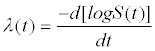and cumulative hazard function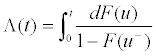. Since we assumed that T and U are independent,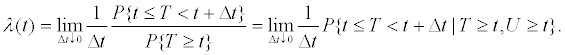(1)

Therefore, if we let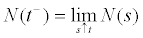then

λ(t)Δt ≈ E[N ((t+Δt)-)-N (t-)|T ≥ t, U ≥ t].

Thus, the hazard function λ(t) represents the conditional average rate of change in N over [t, t+Δt), given that both the censoring and failure time exceed or equal t. For each fixed t,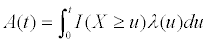is a random variable commonly referred to as the integrated intensity process which approximates the number of jumps by N over (0, t]. Let,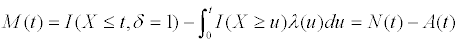Notice that E[N(t)]=E[A(t)] (see (7.6) in Appendix B). Furthermore, suppose that Fs=σ{N (u), I (X ≤ u, δ=0) : 0 ≤ u ≤ s} is the filtration for the process M (t) and Fs- is the information in N(u) and I (X ≤ u, δ=0) up to, but not including time s. Both {X<s} and {X ≥ s} are Fs-–measurable since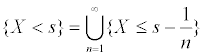. Furthermore, dN(s) is a Bernoulli random variable such that

E[dN (s)|Fs-]=I (X ≥ s)λ(s)ds=dA(s).                     (2)

When we have independent censoring it follows that P {s ≤ T<s+ds|T ≥ s}=P {s ≤ T<s+ds|T ≥ s, U ≥ s} and E[dA(s)|Fs-]=dA(s) (see (7.7) in Appendix B). Therefore, the change in M(t)=N (t)-A(t) over an infinitesimal interval (s-ds, s], dM (s)=dN (s)-dA(s) has expectation 0 given Fs-. Therefore, M is a martingale with respect to {Fs}.

#### Nelson-Aalen Estimator

Suppose time-to-failure data are collected over a finite interval [0, τ] on n subjects with independent failure times. The Nelson-Aalen estimator is a non-parametric estimator of their common cumulative hazard function, Λ(t), in the presence of right censoring. Suppose Ti and Ui are the failure and censoring times and Ni={Ni(t), t ≥ 0} the observed counting process for the ith subject. Let {Yi(t), t ≥ 0} denote a process such that Yi(t)=1 if and only if the ith subject is uncensored and has survived at time t-. It is referred as the at risk process and assumed left-continuous. For each t>0, let Ft-=σ{Ni(u), Yi(u), i=1, ..., n; 0 ≤ u ≤ t} denote the filtration up to, but not including t. Let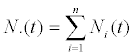and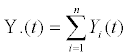denote the aggregate processes that count the numbers of total failures and at risk in the interval (0, t], respectively. Furthermore, let J (t)=I{Y.(t)>0} indicate whether at least one subject is at risk at time t and suppose that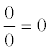. The Nelson-Aalen estimator follows as(3)

Asymptotic Uniform Consistency of the Nelson-Aalen estimator

In this section, we demonstrate how to prove that (3) is an uniformly consistent estimator of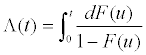, for continuous distribution function, F (t)=P {T ≤ t}. Note that by assuming continuous time we have assumed that no two of the counting processes N1(t), ..., Nn(t) jump at the same moment. Theorem 7.4, known as Lenglart’s Inequality, and related Corollary 7.5 are used to produce the result (Fleming and Harrington, 1991). Two regularity conditions are necessary. First, inft(0,τ]Y.(t) → ∞ in probability as n → ∞. This implies that the number of subjects at risk at each time point becomes large for large n. Second, Λ(τ)<∞, thus, F (t)<1, ∀t ∈ [0, τ ].

First notice,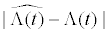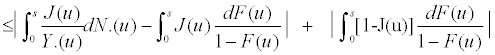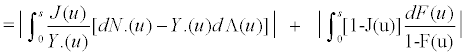(see e.g. (7.8) in Appendix B). We demonstrated in Section 2 that Ni(t) has compensator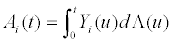. A simple alteration of (7.6) in Appendix B can be used to show that for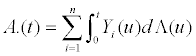,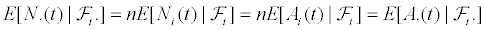Also, since Ai(0)=Λ(0)-Λ(0)=0, for all i=1, ..., n then A.(0)=0. Now we must show that A.(t)<∞ and A.(t) is locally bounded ∀t ∈ [0, τ ]. For all fixed n=1, 2... and all t ∈ [0, τ], it follows that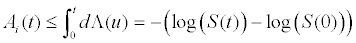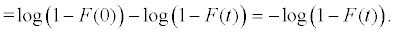The second regularity condition imposes that F(t)<1, ∀t ∈ [0, τ]. Thus, we can conclude that A.(t)<∞, ∀t ∈ [0, τ]. If we consider the localizing sequence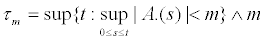for m=1, 2, ..., and stopping process Qm=A.(t ∧ τm), then A. satisfies the conditions for locally bounded. Thus, Theorem 7.3 in Appendix A, which is given by (Fleming and Harrington, 1991), implies that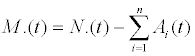a local square integrable martingale. In inequality (7.8) in Appendix B we noted that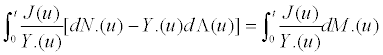. Equation (2) in Section 3 as well as Corollary 7.2 in Appendix A suggest that A.(t) is a predictable process. Therefore, so is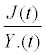. In addition, since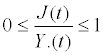for all t ≥ 0 and n>0, it is clearly locally bounded. Corollary 7.5 in Appendix A can be used to show that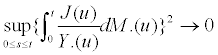, probability where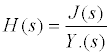. In particular,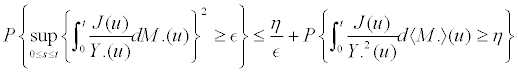Using the variances of increments of M.(t), one can show that,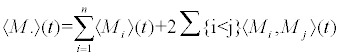(see e.g. (7.9) in Appendix B). The assumption of continuous time implies that for all i ≠ j , Mi, and Mj are orthogonal martingales. In other words, Ni(t) and Nj(t) cannot jump at the exact same moment in time,∀i ≠ j and ∀t ≥ 0. Therefore, we have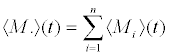Now for any i=1, ..., n and any t>0,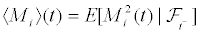because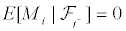Since dNi(t) is a Bernoulli random variable ∀t >0 and ∀i, and dAi(t) is approximately 0 over an infinitesimally small interval,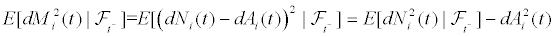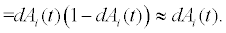Thus, taking the predictable variation process equal to its compensator: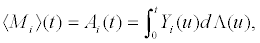it follows from (4) that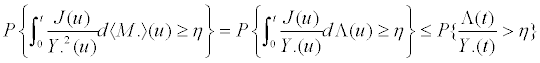From our first regularity condition we have Y.(t) → ∞ in probability, as n → ∞. Therefore,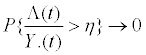in probability for any η>0. Thus, all that is left to show is that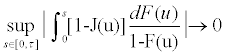probability as n→ ∞. Noting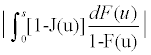is bounded by |Λ(s)-I (Y.(s)>0)Λ(s)| e. g.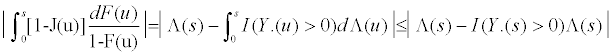From our first regularity condition we can conclude that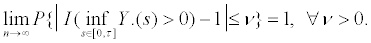Therefore we have,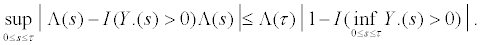Thus, we can use Slutsky’s Theorem to conclude that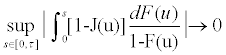in probability as n → ∞. Therefore, the Nelson-Aalen estimator is asymptotically uniformly consistent for the cumulative hazard function under regularity conditions: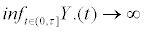in probability as n → ∞ and Λ(τ)<∞.

#### Simulation Study

This section demonstrates the martingale approach for analysis of right-censored failure time data using simulation. Let A1, A2, ... denote independent uniform(0, τ ) random variables where τ is a known constant, and let xi(t)=I (Ai <t), i=1, ..., n. Ni(t) counts observed failures for the ith subject observed over the interval (0, τi], where τi is a constant 0<τi ≤ τ , i=1, ..., n. We assume that the intensity function of Ni(t) with respect to the filtration Ft=σ{Ni(u), Yi(u+), Xi(u+), i=1, ..., n; 0 ≤ u ≤ t} is

λi(t)=Yi(t) exp[xi(t)β]α,          (5)

for 0<t<τ , i=1, ..., n, and α>0. We simulated 30 realizations of the process [N.(t), 0<t<10] and the corresponding martingale when τ=10, n=10, τi=i, for i=1, ..., 10 and fixed “baseline hazard” α=1. For convenience, we fixed β=1. Let zi=exp(1), if xi=1 and=1 otherwise. We approximated continuous time by partitioning [0, 10) into disjoint intervals, tjof length dt=0.1. Now it follows that E[dNi(tj)]=ziαdt at each tj ∈ [0, τ ). For each subject, the process was simulated by iterating through each time interval tj within each subject. At each tj we draw a single sample, dNi(tj), from the Poisson density with rate parameter E[dNi(tj)]. If dNi(tj)>0, then we have observed the failure time for the ith subject to be tj. Therefore, we set counting processes Ni(k)=1 and Yi(k)=0, ∀tj ≤ k ≤ τ. Furthermore, the aggregated counting process N.(k)=N.(k)+1, ∀tj ≤ k ≤ τ.

Figure 1 provides the resulting expected and simulated mean aggregated counting process N.(t) (right panel) as well as the simulated mean martingale (left panel). As expected, The simulated mean aggregated counting process N.(t) mirrors closely its expectation. Further-more, the corresponding simulated mean aggregated martingale illustrates a process that has no random drift.

Figure 1: Results for the simulated mean aggregated counting process N.(t) and expectation (right) and the corresponding simulated mean martingale (left).

#### Discussion

Martingale characterizations play a critical role in the study of large sample properties of the Nelson-Aalen and thereby Kaplan- Meier estimators. This article reviewed martin- gale theory and its role in demonstrating that the Nelson-Aalen estimator is asymptotically uniformly consistent for the cumulative hazard function for rightcensored continuous time- to-failure data and demonstrates its application using simulation.

#### Acknowledgement

The author was partially supported by NIH/NCI grants R01CA157458 and P30CA016672.

#### References

Select your language of interest to view the total content in your interested language

### Article Usage

• Total views: 12209
• [From(publication date):
June-2015 - Aug 25, 2019]
• Breakdown by view type
• HTML page views : 8403
• PDF downloads : 3806

## Post your commentCan't read the image? click here to refresh
###### Peer Reviewed Journals

Make the best use of Scientific Research and information from our 700 + peer reviewed, Open Access Journals

International Conferences 2019-20

Meet Inspiring Speakers and Experts at our 3000+ Global Annual Meetings

Top